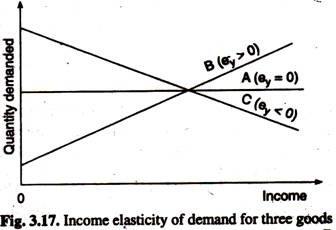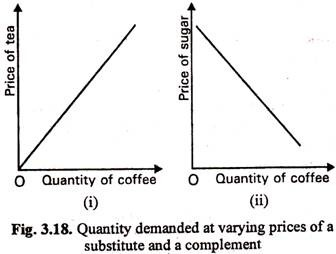# 2 Major Types of Elasticity (With Formula)

The following points highlight the two major types of elasticity. The two types are: A. Income Elasticity B. Cross-Elasticity of Demand.

#### A. Income Elasticity of Demand:

Income elasticity of demand is the degree to which the demand for a commodity responds to a change in income.

The coefficient of income elasticity may be measured by using the following formula:

ey = Proportionate change in quantity demanded/Proportionate change in income.

Price elasticity of demand is always negative. Income elasticity, however, may be positive or negative. For most goods it will be positive, i.e., if income rises, demand for the commodity also rises, whereas, if income falls, demand for the commodity falls.

But, for inferior goods, income elasticity will be negative, i.e., if income rises, demand for an inferior good will fall, whereas, if income falls, demand for an inferior good will rise. Thus with a product like margarine, if income rises, people might now be able to afford butter instead and the demand for margarine will fall.The boundary between positive and negative, income elasticity is zero income elasticity, corresponding to the case where a change in income leaves quantity demanded unchanged. Fig. 3.17 shows the relationship between income and quantity demanded for the three types of goods i.e., A, B and C.

#### B. Cross-Elasticity of Demand:

Cross-elasticity of demand is the degree to which the demand for one commodity res­ponds to a change in the price of another commodity.

The formula for measuring the coefficient of cross elasticity of demand is:

ec = Proportionate change in demand for A/Proportionate change in price of B

Cross elasticity may be positive or negative, depending on the relationship between the two commodities. If the commodities are substitutes, cross elasticity will be positive, i.e., a rise in the price of the first commodity will cause an increase in the demand for the other commodity. For example, a 5 per cent rise in the price of tea might result in a 6 per cent increase in the demand for coffee, in which case cross elasticity is (6/100) (5/100) = 1.2.

If the commodities are complements, cross- elasticity will be negative, i.e., a rise in the price of one commodity will cause a fall in the demand for the other. For example, if the price of sugar rises, less sugar will be bought and there will be a fall in the demand for coffee also. If a 10 percent rise in the price of sugar results in a fall of 8 per cent in the demand for coffee, cross elasticity is (-8/100)/ (10/100) = – 0.8.

In fact, the value of cross elasticity of demand may vary between minus infinity and plus infinity. Fig. 3.18(i) shows that cross- elasticity of demand for substitute goods is positive and for complementary goods it is negative. The cross-elasticity of demand in case of tea and coffee will be positive because a fall in the price of tea would lead consumers to substitute it for coffee.

The relationship would be as in Fig. 3.18(i). As the price of tea falls, the demand for coffee falls, too. In contrast, the cross-elasticity of demand for coffee with respect to the price of sugar is likely to be negative. The reason is simple: a fall in the price of sugar would lead to an increase in the demand for coffee, as shown in Fig. 3.18(ii). The reason is simple each cup of coffee will now cost less than before.Cross-elasticity is positive in case of substitute goods like tea and coffee, is almost zero in case of unrelated goods such as butter and tea and is negative in case of compli­mentary goods such as cars and petrol. In the first case a rise in the price of one will bring about an increase in the demand for another. In contrast, a rise in the price of petrol will lead to a fall in the demand for cars.

In fact, the value of Ee may vary from minus infinity to plus infinity. Goods which are close substitutes (like Limca and Campa Cola) or complements (kerosene stoves and kerosene) will tend to show a fairly high degree of Ec. Conversely, when there is little, if any, relation between goods then the Ec will be near to zero.